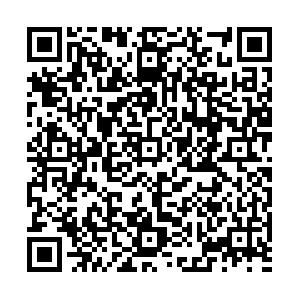# Analysis of the 1S and 2S states of ΛQ and ΞQ with QCD sum rules• In this article, we study the ground states and the first radial excited states of the flavor antitriplet heavy baryon states $\Lambda_Q$and $\Xi_Q$with the spin-parity $J^P={1\over 2}^{+}$by carrying out operator product expansion up to vacuum condensates of dimension $10$in a consistent way. We observe for the first time that the higher dimensional vacuum condensates play an important role, and obtain very stable QCD sum rules with variations of the Borel parameters for the heavy baryon states. The predicted masses $6.08\pm0.09\,{\rm{GeV}}$, $2.78\pm0.08\,{\rm{GeV}}$, and $2.96\pm0.09\,{\rm{GeV}}$for the first radial excited states $\Lambda_b(2{{S}})$, $\Lambda_c(2{{S}})$, and $\Xi_c(2{{S}})$, respectively, are in excellent agreement with the experimental data and support assigning $\Lambda_b(6072)$, $\Lambda_c(2765)$, and $\Xi_c(2980/2970)$to be the first radial excited states of $\Lambda_b$, $\Lambda_c$, and $\Xi_c$, respectively. The predicted mass $6.24\pm0.07\,{\rm{GeV}}$for $\Xi_b(2{{S}})$can be confirmed using experimental data in the future.
••Get Citation
Zhi-Gang Wang and Hui-Juan Wang. Analysis of the 1S and 2S states of the ΛQ and ΞQ with the QCD[J]. Chinese Physics C. doi: 10.1088/1674-1137/abc1d3
Zhi-Gang Wang and Hui-Juan Wang. Analysis of the 1S and 2S states of the ΛQ and ΞQ with the QCD[J]. Chinese Physics C.Milestone
Article Metric

Article Views(122)
Cited by(0)
Policy on re-use
To reuse of Open Access content published by CPC, for content published under the terms of the Creative Commons Attribution 3.0 license (“CC CY”), the users don’t need to request permission to copy, distribute and display the final published version of the article and to create derivative works, subject to appropriate attribution.
###### 通讯作者: 陈斌, bchen63@163.com
• 1.

沈阳化工大学材料科学与工程学院 沈阳 110142

Title:
Email:

## Analysis of the 1S and 2S states of ΛQ and ΞQ with QCD sum rules

• Department of Physics, North China Electric Power University, Baoding 071003, China

Abstract: In this article, we study the ground states and the first radial excited states of the flavor antitriplet heavy baryon states $\Lambda_Q$and $\Xi_Q$with the spin-parity $J^P={1\over 2}^{+}$by carrying out operator product expansion up to vacuum condensates of dimension $10$in a consistent way. We observe for the first time that the higher dimensional vacuum condensates play an important role, and obtain very stable QCD sum rules with variations of the Borel parameters for the heavy baryon states. The predicted masses $6.08\pm0.09\,{\rm{GeV}}$, $2.78\pm0.08\,{\rm{GeV}}$, and $2.96\pm0.09\,{\rm{GeV}}$for the first radial excited states $\Lambda_b(2{{S}})$, $\Lambda_c(2{{S}})$, and $\Xi_c(2{{S}})$, respectively, are in excellent agreement with the experimental data and support assigning $\Lambda_b(6072)$, $\Lambda_c(2765)$, and $\Xi_c(2980/2970)$to be the first radial excited states of $\Lambda_b$, $\Lambda_c$, and $\Xi_c$, respectively. The predicted mass $6.24\pm0.07\,{\rm{GeV}}$for $\Xi_b(2{{S}})$can be confirmed using experimental data in the future.

### HTMLII.   QCD SUM RULES FOR THE ${\Lambda_Q(1S,2S)}$ AND ${\Xi_Q(1S,2S)}$III.   NUMERICAL RESULTS AND DISCUSSIONS
Reference (55)
PDF查看关注分享

Top

### 目录/DownLoad:  Full-Size Img  PowerPoint